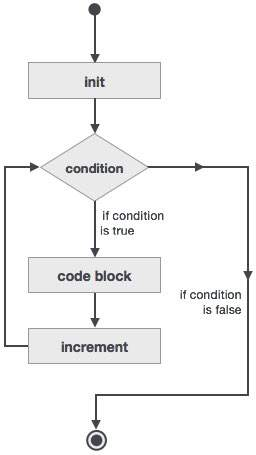# Loops adds +1 to #i?

Hi I have never noticed this before, but now it bothers me and I’d like to know the reasoning behind it.

When I run a loop like this:

``````+PROG TEMPLATE
loop#i 5
prt#i
endloop
prt#i
END
``````

I get the following output.

``````   1 +PROG TEMPLATE
3 \$ Job : PC97065:003385
4     LOOP#I 5
5         PRT#I
6     ENDLOOP
---- CADINT VARIABLE I               (     0) =       0.000
---- CADINT VARIABLE I               (     0) =       1.000
---- CADINT VARIABLE I               (     0) =       2.000
---- CADINT VARIABLE I               (     0) =       3.000
---- CADINT VARIABLE I               (     0) =       4.000
7     PRT#I
---- CADINT VARIABLE I               (     0) =       5.000
8 END
``````

Why, does Sofistik add 1 to #i outside the loop?

If I run a similar piece of code in e.g. Python I get the following:

``````for i in range(5):
print(i)
print(i)
``````

Output:

``````0
1
2
3
4
4
``````

i.e. i is the same value outside the loop as it in inside the loop.

Soooo. Does anyone know why sofistik doing this counterintuitive addition?
Is it a bug or a feature?

Hello

The SOFiSTiK loop is based on the Fortran DO-loops.(source: Fortran - Do Loop Construct)

So the increment is updated after the code block.
In your example, this means that the variable is incremented to 5 after the last loop iteration.

The range() function in python is working in a different way. The last value 5 is only an argument in the range() function. So range(5) greates the vector [0,1,2,3,4].
Now the Python for loop executes the loop for each entry of the vector.

If you want to recreate the same program behaviour in python, you have to use the following code.

``````i = 0
while i < 5:
print(i)
i += 1

print(i)
``````

Further information:

Best regards
Frederik Höller
Your SOFiSTiK Support Team

1 Like

You can use it measure vector length, i.e.

``````Let#A 1,2,3,4,5
Loop#1 A
EndLoop
Let#L #1 \$ #L=5
``````

Since there isn’t any other way to measure vector length in cadinp, I’d call it a feature.

1 Like

You can use it measure vector length, i.e.

``````Let#A 1,2,3,4,5
Loop#1 A
EndLoop
Let#L #1 \$ #L=5
``````

Since there isn’t any other way to measure vector length in cadinp, I’d call it a feature.

Now that you mention it, I have actually used that to measure lengths quite a bitGreat to know. Thank you very much!